# Benchmarking Scala

Microbenchmarking is controversial as the following links show:

Nevertheless I did write a little micro-benchmarking framework in Scala so I could experiment with the Scala language and libraries.

It allows to run little code snippets such as:

```object LoopExample extends ImageReport {
def main(args: Array[String]) {
lineChart("Loops", 0 to 2, 0 to 100000 by 10000,
new FunBenchmarks[Int, Int] {
prepare {
count => count
}
run("for loop", "An empty for loop.") { count =>
for (i <- 0 to count) {
}
}
run("while loop", "A while loop that counts in a variable without returning a result.") { count =>
var i = 0
while (i < count) {
i += 1
}
}
})
}
```

This produced the image used in the last blog Scala for (i <- 0 to n) : nice but slow: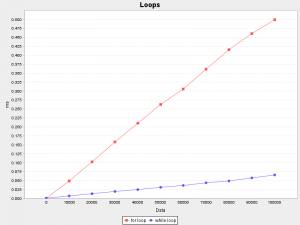The framework also allows to create an HTML report containing multiple benchmarks suites.

Let's have a look at some of the more interesting results.

Note: All micro-benchmarking results should always be interpreted with a very critical mindset. Many things can go wrong when measuring a single operation over and over again. It is very easy to screw up and become meaningless results.
If you want to analyze a particular benchmark in more details, follow the details link at the end of the suite chapter. It will show a detailed statistical analysis of this particular benchmark suite.

## Loops

```        run("for loop", "An empty for loop.") { count =>
for (i <- 0 to count) {
}
}
run("for loop result", "A for loop that accumulates a result in a variable.") { count =>
var result = 0
for (i <- 0 to count) {
result += i
}
result
}
run("while loop", "A while loop that counts in a variable without returning a result.") { count =>
var i = 0
while (i < count) {
i += 1
}
}
run("while loop result", "A while loop that accumulates a result in a variable.") { count =>
var result = 0
var i = 0
while (i < count) {
result += i
i += 1
}
result
}
run("do while loop", "A do-while loop that counts in a variable without returning a result.") { count =>
var i = 0
do {
i += 1
} while (i <= count)
}
run("do while loop result", "A do-while loop that accumulates a result in a variable.") { count =>
var result = 0
var i = 0
do {
result += i
i += 1
} while (i <= count)
result
}
```

As already discussed in another blog entry, loops in Scala perform surprisingly different: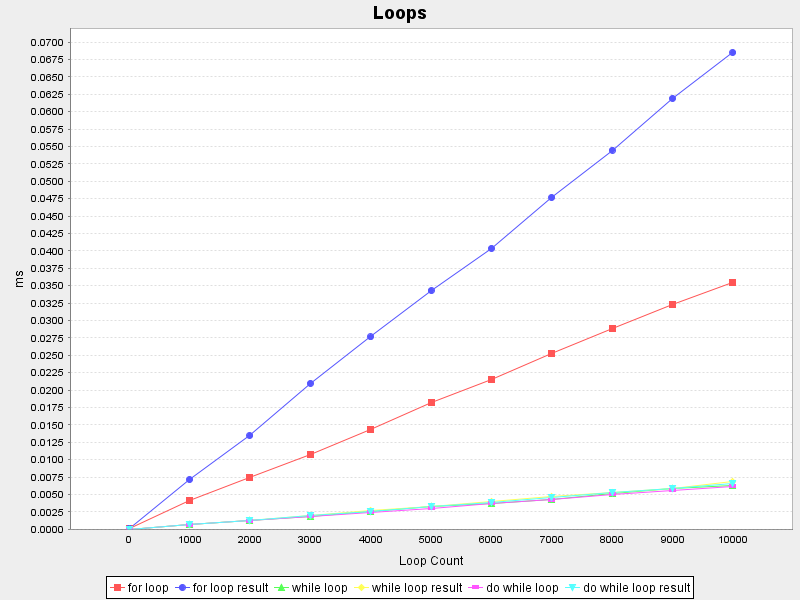The `for (i <- 1 to count)` loop is significantly slower than `while (i < count)`.

## Arithmetic

Not really a surprise, but `BigDecimal` arithmetic is very slow compared to `double` arithmetic (on both charts the red line is the reference benchmark that executes in the same time).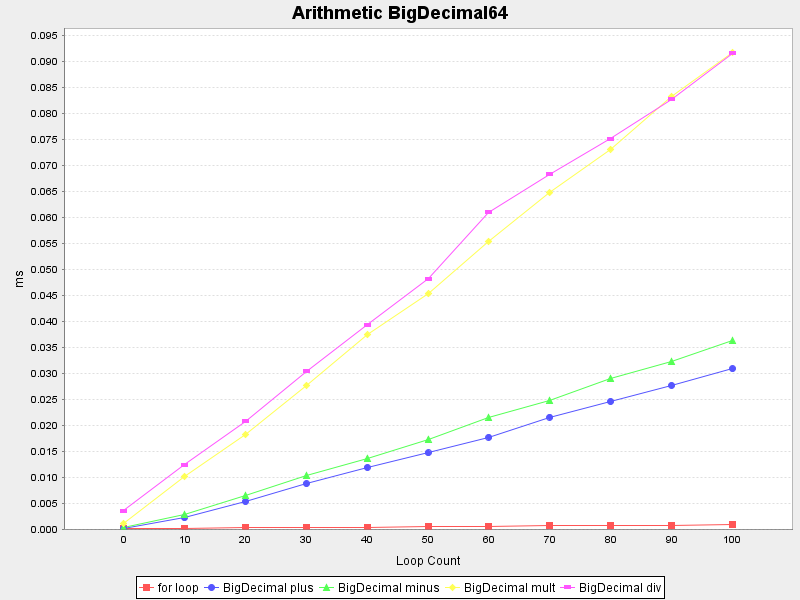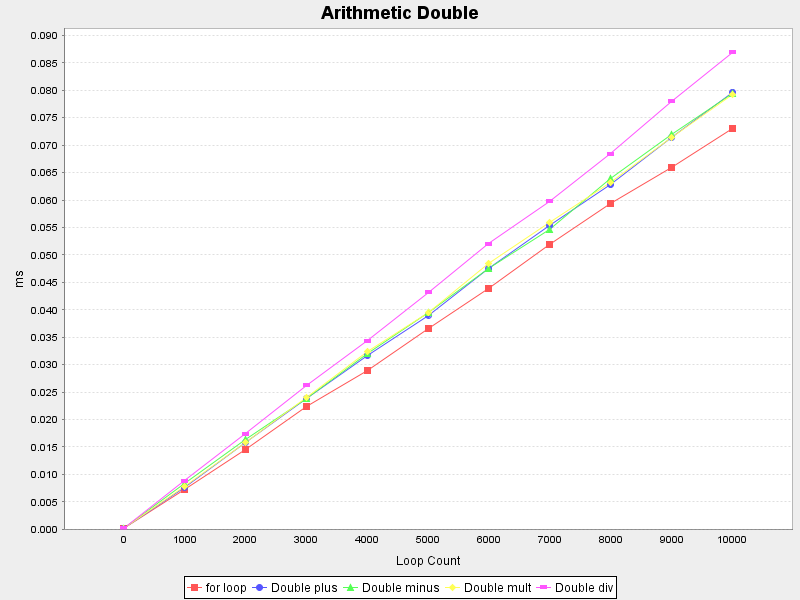## Casts

I doubted the performance of the Scala way of casting a reference, so I compared it with the Java-like cast method:

```        var any: Any = "Hello"
var result: String = _

run("asInstanceOf", "Casts a value using asInstanceOf.") { count =>
for (i <- 0 to count) {
result = any.asInstanceOf[String]
}
}
run("match case", "Casts a value using pattern matching with the type.") { count =>
for (i <- 0 to count) {
result = any match {
case s: String => s
case _ => throw new IllegalArgumentException
}
}
}
```

Happily they perform practically the same: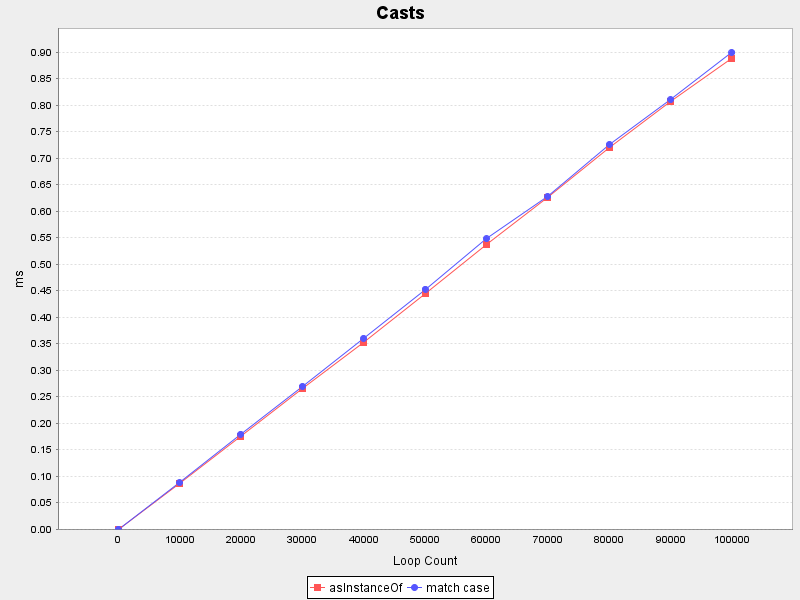## Immutable Map

I am really fond of immutable maps, they are easy to reason use and perform very well.

```        run("contains true", "Checks that a map really contains a value.") { map =>
map.contains(0)
}
run("contains false", "Checks that a map really does not contain a value.") { map =>
map.contains(-999)
}
run("+", "Adds a new entry to a map.") { map =>
map + (-1 -> "X-1")
}
run("-", "Removes an existing entry from a map.") { map =>
map - 0
}
```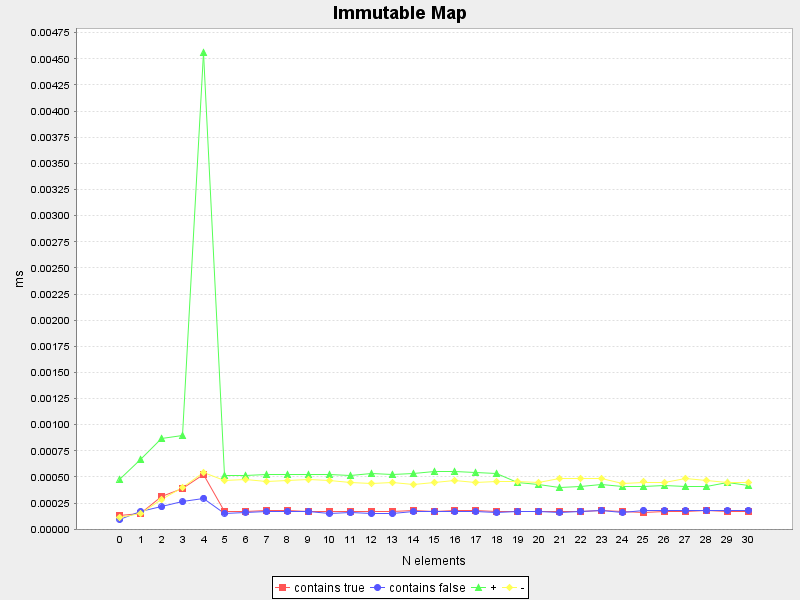The immutable maps with size 0 to 4 are special classes that store the key/value pairs directly in dedicated fields (testing sequentially) - therefore we see linear behaviour there.

The strong peak when adding another key/value pair to an immutable map with a size of 4 is probably because it switches to the normal `scala.collection.immutable.HashMap` (and creating 4 tuples) after testing all keys:

```    // Implementation detail of scala.collection.immutable.Map4
override def updated [B1 >: B] (key: A, value: B1): Map[A, B1] =
if (key == key1)      new Map4(key1, value, key2, value2, key3, value3, key4, value4)
else if (key == key2) new Map4(key1, value1, key2, value, key3, value3, key4, value4)
else if (key == key3) new Map4(key1, value1, key2, value2, key3, value, key4, value4)
else if (key == key4) new Map4(key1, value1, key2, value2, key3, value3, key4, value)
else new HashMap + ((key1, value1), (key2, value2), (key3, value3), (key4, value4), (key, value))
def + [B1 >: B](kv: (A, B1)): Map[A, B1] = updated(kv._1, kv._2)
```

## HashMap

The standard Java `java.util.HashMap` is also interesting for Java programmers.

```        run("contains true", "Checks that a map really contains a value.") { map =>
map.containsKey(0)
}
run("contains false", "Checks that a map really does not contain a value.") { map =>
map.containsKey(-999)
}
run("size", "Calculates the size of a map.") { map =>
map.size()
}
```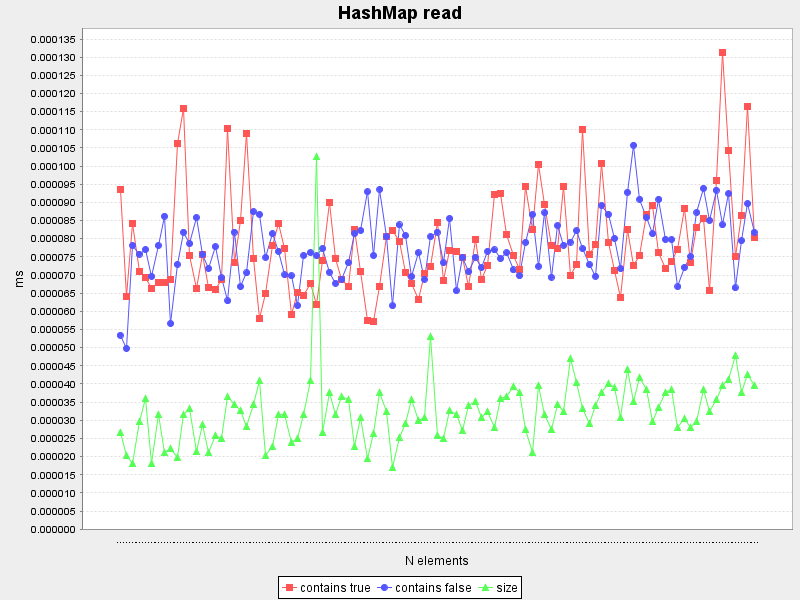At first glance `containsKey` and `size()` seem to be constant (as expected), but to be sure an additional benchmark with a larger n up to a 1000 was added: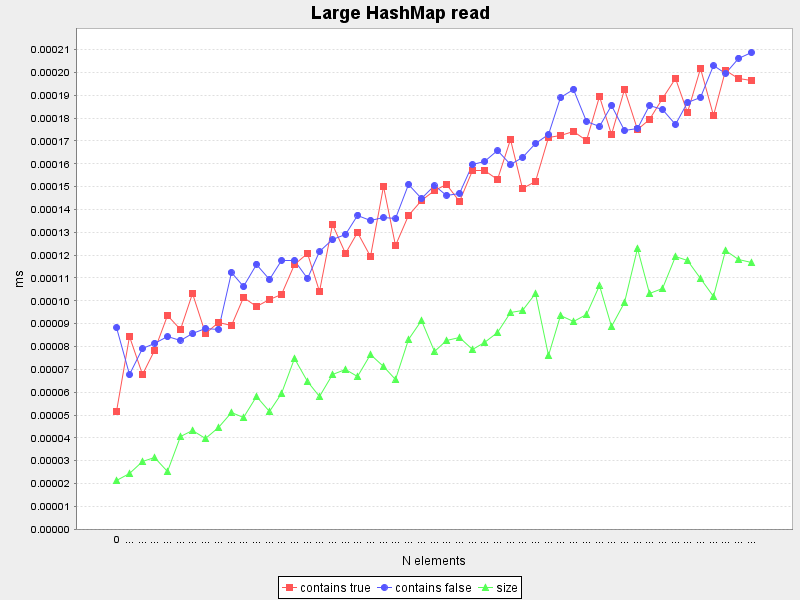Surprisingly all measured methods grow slowly with increasing size (contrary to the expected constant time behaviour) - this needs to be analyzed in detail.

A look at the details of this suite shows that actually all measured elapsed times are either 0.00000 ms or 0.00038 ms and the apparent increase with growing size is purely a statistical side effect. Obviously this benchmark is at the low end of measurement accuracy.

The measured write operations are:

```        run("put", "Adds a new entry to a map.") { map =>
map.put(-1, "X-1")
}
run("remove", "Removes an existing entry from a map.") { map =>
map.remove(0)
}
run("clear", "Removes all entries from a map.") { map =>
map.clear()
}
```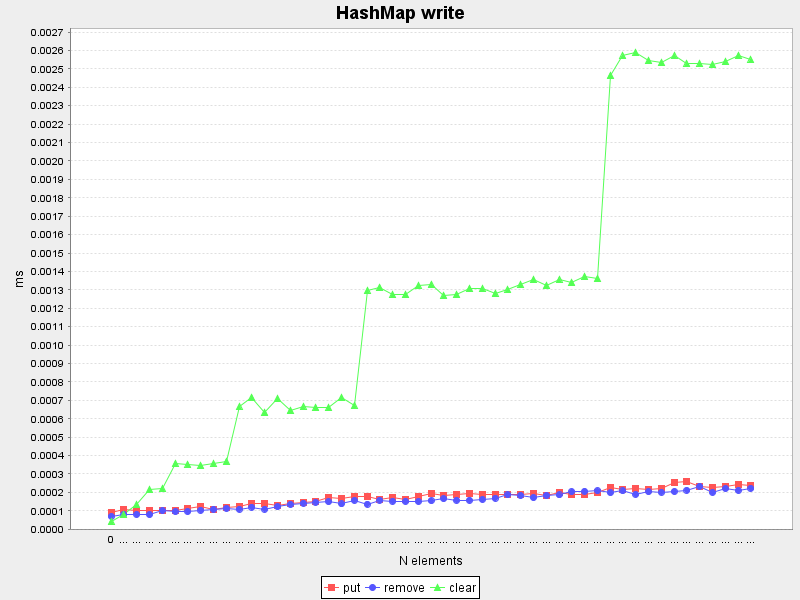`put()` and `remove()` are reasonably constant, albeit they grow very slowly with increasing size.

I was very surprised to see that the `clear()` method is not constant time. A quick look at the implementation shows that it is linear with the number of entries.

```    // Implementation of java.util.HashMap.clear()
public void clear() {
modCount++;
Entry[] tab = table;
for (int i = 0; i < tab.length; i++)
tab[i] = null;
size = 0;
}
```

## ConcurrentHashMap

The behaviour of `java.util.concurrent.ConcurrentHashMap` is very similar to `HashMap` (slightly slower).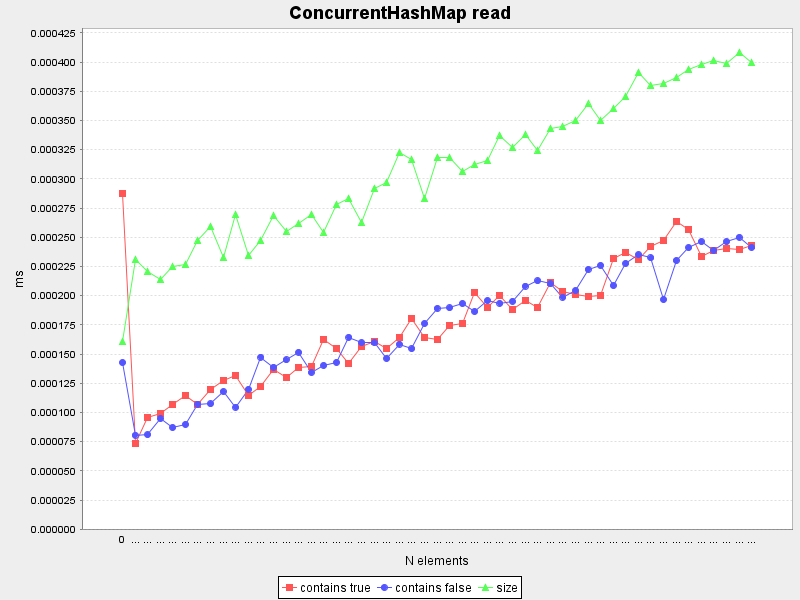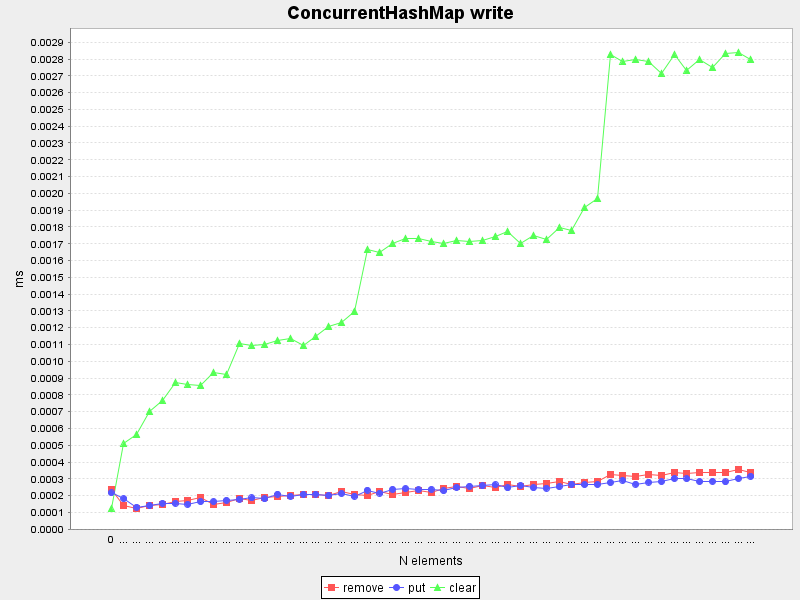## Pattern Matching

Pattern matching is a very nice and powerful feature of Scala.

This benchmark tests only the simplest case of pattern matching and compares them with a comparable if-else cascade.

```        run("match 1", "Matches the 1st pattern with a literal integer value.") { seq =>
for (value <- seq) {
value match {
case 1 => "one"
case _ => "anything"
}
}
}
run("if 1", "Matches the 1st if in an if-else cascade with integer values.") { seq =>
for (value <- seq) {
if (value == 1) "one"
else "anything"
}
}
run("match 5", "Matches the 5th pattern with a literal integer value.") { seq =>
for (value <- seq) {
value match {
case 1 => "one"
case 2 => "two"
case 3 => "three"
case 4 => "four"
case 5 => "five"
case _ => "anything"
}
}
}
run("if 5", "Matches the 5th if in an if-else cascade with integer values.") { seq =>
for (value <- seq) {
if (value == 1) "one"
else if (value == 2) "two"
else if (value == 3) "three"
else if (value == 4) "four"
else if (value == 5) "five"
else "anything"
}
}
```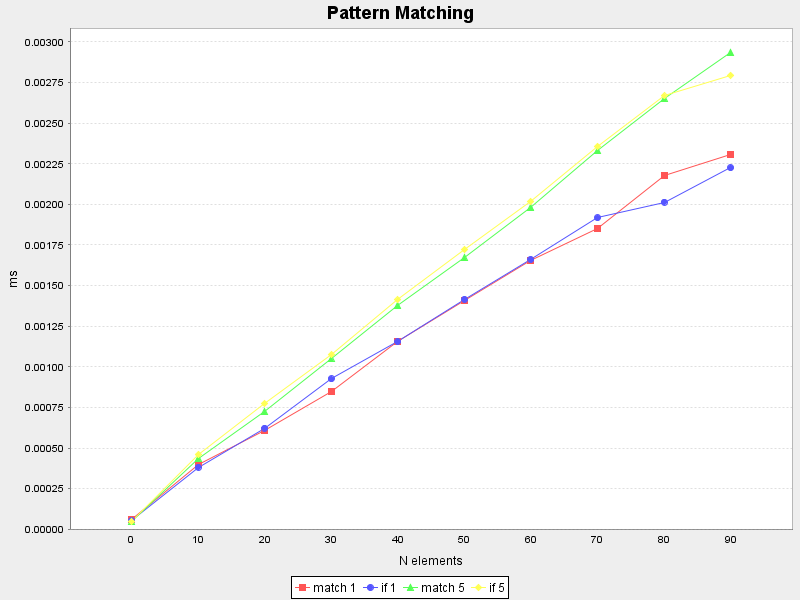As you see, simple pattern matching and the if-else cascade have comparable speed.

This entry was posted in Scala. Bookmark the permalink.